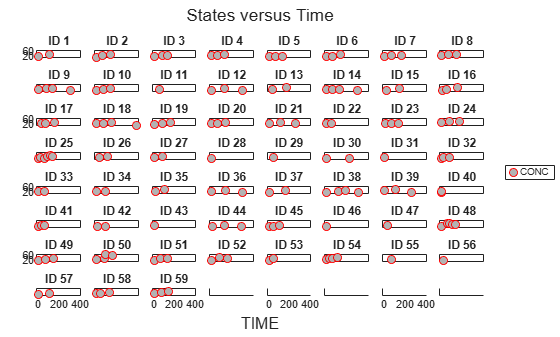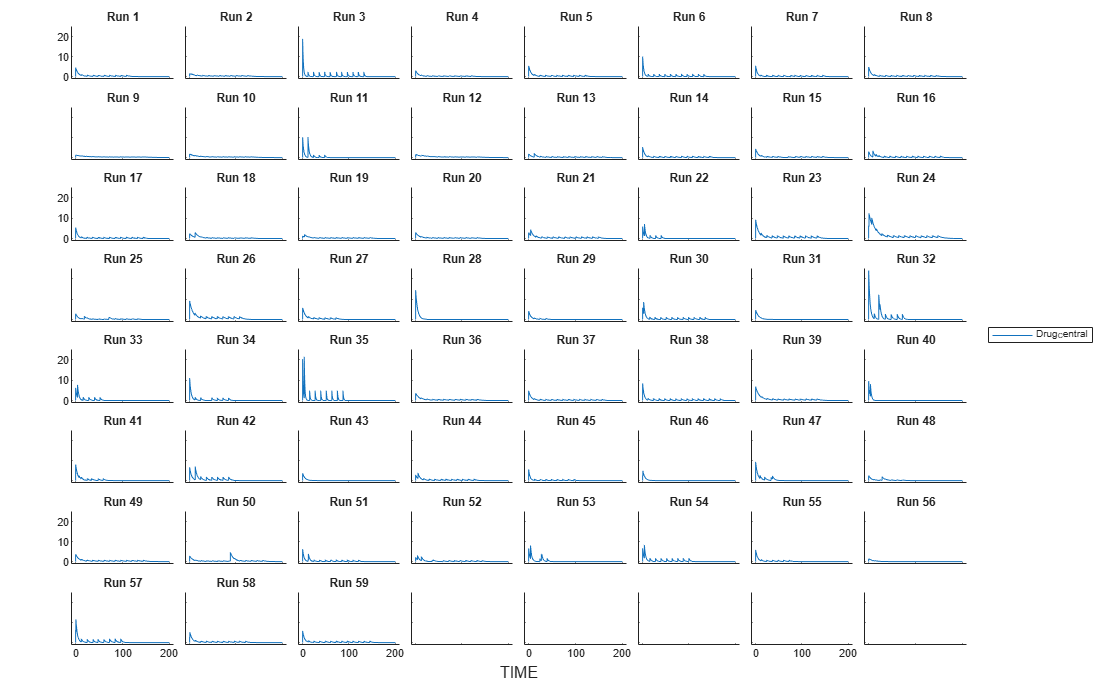# sbiosampleparameters

Generate parameters by sampling covariate model (requires Statistics and Machine Learning Toolbox software)

## Syntax

``phi = sbiosampleparameters(covexpr,thetas,omega,ds)``
``phi = sbiosampleparameters(covexpr,thetas,omega,n)``
``````[phi,covmodel] = sbiosampleparameters(_)``````

## Description

example

````phi = sbiosampleparameters(covexpr,thetas,omega,ds)` generates a matrix `phi` containing sampled parameter values using the covariate model specified by the covariate expression `covexpr`, fixed effects `thetas`, covariance matrix `omega`, and covariate data `ds`.```

example

````phi = sbiosampleparameters(covexpr,thetas,omega,n)` uses a scalar `n` that specifies the number of rows in `phi` when the parameters are not dependent on any covariate.```

example

``````[phi,covmodel] = sbiosampleparameters(_)``` returns a matrix `phi` and a covariate model object `covmodel` using any of the input arguments from previous syntaxes.```

## Examples

collapse all

This example uses data collected on 59 preterm infants given phenobarbital during the first 16 days after birth. Each infant received an initial dose followed by one or more sustaining doses by intravenous bolus administration. A total of between 1 and 6 concentration measurements were obtained from each infant at times other than dose times, for a total of 155 measurements. Infant weights and APGAR scores (a measure of newborn health) were also recorded. Data was described in , a study funded by the NIH/NIBIB grant P41-EB01975.

`load pheno.mat ds`

Visualize the data.

```t = sbiotrellis(ds,'ID','TIME','CONC','marker','o','markerfacecolor',[.7 .7 .7],'markeredgecolor','r','linestyle','none'); t.plottitle = 'States versus Time';```Create a one-compartment PK model with bolus dosing and linear clearance to model such data.

```pkmd = PKModelDesign; pkmd.addCompartment('Central','DosingType','Bolus','EliminationType','linear-clearance',... 'HasResponseVariable',true,'HasLag',false); onecomp = pkmd.construct;```

Suppose there is a correlation between the volume of the central compartment (`Central`) and the weight of infants. You can define this parameter-covariate relationship using a covariate model that can be described as

$\mathrm{log}\left({V}_{i}\right)={\theta }_{V}+{\theta }_{V}{WEIGHT}}\ast WEIGH{T}_{i}+{\eta }_{V,i}$,

where, for each ith infant, `V` is the volume, θs (thetas) are fixed effects, η (eta) represents random effects, and `WEIGHT` is the covariate.

```covM = CovariateModel; covM.Expression = {'Central = exp(theta1+theta2*WEIGHT+eta1)'};```

Define the fixed and random effects. The column names of each table must have the names of fixed effects and random effects, respectively.

```thetas = table(1.4,0.05,'VariableNames',{'theta1','theta2'}); eta1 = table(0.2,'VariableNames',{'eta1'});```

Change the group label ID to GROUP as required by the `sbiosampleparameters` function.

`ds.Properties.VariableNames{'ID'} = 'GROUP';`

Generate parameter values for the volumes of central compartments Central based on the covariate model for all infants in the data set.

`phi = sbiosampleparameters(covM.Expression,thetas,eta1,ds);`

You can then simulate the model using the sampled parameter values. For convenience, use the function-like interface provided by a SimFunction object.

First, construct a SimFunction object using the createSimFunction method, specifying the volume (Central) as the parameter, and the drug concentration in the compartment (Drug_Central) as the output of the SimFunction object, and the dosed species.

`f = createSimFunction(onecomp,covM.ParameterNames,'Drug_Central','Drug_Central');`

The data set ds contains dosing information for each infant, and the groupedData object provides a convenient way to extract such dosing information. Convert ds to a groupedData object and extract dosing information.

```grpData = groupedData(ds); doses = createDoses(grpData,'DOSE');```

Simulate the model using the sampled parameter values from phi and the extracted dosing information of each infant, and plot the results. The ith run uses the ith parameter value in phi and dosing information of the ith infant.

```t = sbiotrellis(f(phi,200,doses.getTable),[],'TIME','Drug_Central'); % Resize the figure. t.hFig.Position(:) = [100 100 1280 800];```## Input Arguments

collapse all

Covariate expressions, specified as a cell array of character vectors or string vector that defines the parameter-covariate relationships.

If a model component name or covariate name is not a valid MATLAB® variable name, surround it by square brackets when referring to it in the expression. For example, if the name of a species is DNA polymerase+, write `[DNA polymerase+]`. If a covariate name itself contains square brackets, you cannot use it in the expression.

See `CovariateModel object` to learn more about covariate expressions.

Fixed effects, specified as a table, dataset, or numeric vector containing values for fixed effect parameters defined in the covariate expressions `covexpr`. Fixed effect parameter names must start with `'theta'`.

• If `thetas` is a table, `thetas.Properties.VariableNames` must match the names of the fixed effects.

For example, suppose that you have three thetas: ```thetaOne = 0.1```, ```theta2 = 0.2```, and ```theta3 = 0.3```. You can create the corresponding table.

```thetas = table(0.1,0.2,0.3); thetas.Properties.VariableNames = {'thetaOne','theta2','theta3'}```
```thetas = 1×3 table thetaOne theta2 theta3 ________ ______ ______ 0.1 0.2 0.3 ```

• If `thetas` is a dataset, `thetas.Properties.VarNames` must match the names of the fixed effects.

• If `thetas` is a numeric vector, the order of the values in the vector must be the same ascending ASCII dictionary order as the fixed effect names.

Use the `sort` function to sort a cell array of character vectors to see the order.

`sort({'thetaOne','theta2','theta3'})`
```ans = 1×3 cell array {'theta2'} {'theta3'} {'thetaOne'}```

Then specify the value of each theta in the same order.

`thetas = [0.2 0.3 0.1];`

Covariance matrix of random effects, specified as a table, dataset, or matrix. Random effect parameter names must start with `'eta'`.

• If `omega` is a table, `omega.Properties.VariableNames` must match the names of the random effects. Specifying the row names (`RowNames`) is optional, but if you do, they must also match the names of random effects.

Suppose that you want to define a diagonal covariance matrix with three random effect parameters `eta1`, `eta2`, and `eta3` with the values `0.1`, `0.2`, and `0.3`, respectively.

$\left[\begin{array}{l}Cov\left({\eta }_{1},{\eta }_{1}\right)\text{​}\text{​}\text{​}\text{​}\text{\hspace{0.17em}}\text{\hspace{0.17em}}\text{\hspace{0.17em}}\text{\hspace{0.17em}}\text{\hspace{0.17em}}Cov\left(\eta {}_{1},{\eta }_{2}\right)\text{\hspace{0.17em}}\text{\hspace{0.17em}}\text{\hspace{0.17em}}\text{\hspace{0.17em}}\text{\hspace{0.17em}}Cov\left({\eta }_{1},{\eta }_{3}\right)\\ Cov\left({\eta }_{2},{\eta }_{1}\right)\text{\hspace{0.17em}}\text{\hspace{0.17em}}\text{\hspace{0.17em}}\text{\hspace{0.17em}}\text{\hspace{0.17em}}Cov\left({\eta }_{2},{\eta }_{2}\right)\text{\hspace{0.17em}}\text{\hspace{0.17em}}\text{\hspace{0.17em}}\text{\hspace{0.17em}}\text{\hspace{0.17em}}Cov\left({\eta }_{2},{\eta }_{3}\right)\\ Cov\left({\eta }_{3},{\eta }_{1}\right)\text{\hspace{0.17em}}\text{\hspace{0.17em}}\text{\hspace{0.17em}}\text{\hspace{0.17em}}\text{\hspace{0.17em}}Cov\left({\eta }_{3},{\eta }_{2}\right)\text{\hspace{0.17em}}\text{\hspace{0.17em}}\text{\hspace{0.17em}}\text{\hspace{0.17em}}\text{\hspace{0.17em}}Cov\left({\eta }_{3},{\eta }_{3}\right)\end{array}\right]=\left[\begin{array}{l}eta1\text{\hspace{0.17em}}\text{\hspace{0.17em}}\text{\hspace{0.17em}}\text{\hspace{0.17em}}\text{\hspace{0.17em}}\text{\hspace{0.17em}}0\text{\hspace{0.17em}}\text{\hspace{0.17em}}\text{\hspace{0.17em}}\text{\hspace{0.17em}}\text{\hspace{0.17em}}\text{\hspace{0.17em}}\text{\hspace{0.17em}}\text{\hspace{0.17em}}\text{\hspace{0.17em}}\text{\hspace{0.17em}}\text{\hspace{0.17em}}0\\ 0\text{\hspace{0.17em}}\text{\hspace{0.17em}}\text{\hspace{0.17em}}\text{\hspace{0.17em}}\text{\hspace{0.17em}}\text{\hspace{0.17em}}\text{\hspace{0.17em}}\text{\hspace{0.17em}}\text{\hspace{0.17em}}eta2\text{\hspace{0.17em}}\text{\hspace{0.17em}}\text{\hspace{0.17em}}\text{\hspace{0.17em}}\text{\hspace{0.17em}}\text{\hspace{0.17em}}\text{\hspace{0.17em}}0\\ 0\text{\hspace{0.17em}}\text{\hspace{0.17em}}\text{\hspace{0.17em}}\text{\hspace{0.17em}}\text{\hspace{0.17em}}\text{\hspace{0.17em}}\text{\hspace{0.17em}}\text{\hspace{0.17em}}\text{\hspace{0.17em}}\text{\hspace{0.17em}}\text{\hspace{0.17em}}\text{\hspace{0.17em}}0\text{\hspace{0.17em}}\text{\hspace{0.17em}}\text{\hspace{0.17em}}\text{\hspace{0.17em}}\text{\hspace{0.17em}}\text{\hspace{0.17em}}\text{\hspace{0.17em}}\text{\hspace{0.17em}}\text{\hspace{0.17em}}eta3\end{array}\right]$

You can construct the corresponding table.

```eta1 = [0.1;0;0]; eta2 = [0;0.2;0]; eta3 = [0;0;0.3]; omega = table(eta1,eta2,eta3,'VariableNames',{'eta1','eta2','eta3'})```
```omega = 3×3 table eta1 eta2 eta3 ____ ____ ____ 0.1 0 0 0 0.2 0 0 0 0.3 ```

• If `omega` is a dataset, `omega.Properties.VarNames` must match the names of the random effects. Specifying the row names (`ObsNames`) is optional, but if you do, they must also match the names of random effects.

• If `omega` is a matrix, the rows and columns must have the same ascending ASCII dictionary order as the random effect names.

Use the `sort` function to sort a cell array of character vectors to see the order.

`sort({'eta1','eta2','eta3'})`
```ans = 1×3 cell array {'eta1'} {'eta2'} {'eta3'}```

Covariate data, specified as a dataset or table containing the covariate data for all groups.

`ds` must have a column named `'Group'` or `'GROUP'` specifying the group labels as well as a column each for all covariates used in the covariate model. The column names must match the names of the corresponding covariates used in the covariate expressions.

Number of rows in `phi`, specified as a scalar.

## Output Arguments

collapse all

Sampled parameter values, returned as a matrix of size S-by-P, where `S` is the number of groups specified in `ds` or specified by `n` and `P` is the number of parameters which is equal to the number of elements in `covexpr`.

Covariate model, returned as a `CovariateModel object` which represents the model defined by `covexpr`.

## Compatibility Considerations

expand all

Warns starting in R2018b

 Grasela Jr, T.H., Donn, S.M. (1985) Neonatal population pharmacokinetics of phenobarbital derived from routine clinical data. Dev Pharmacol Ther. 8(6), 374–83.

﻿
##### Support